Test: Structural Analysis- 3

# Test: Structural Analysis- 3

Test Description

## 10 Questions MCQ Test GATE Civil Engineering (CE) 2023 Mock Test Series | Test: Structural Analysis- 3

Test: Structural Analysis- 3 for Civil Engineering (CE) 2023 is part of GATE Civil Engineering (CE) 2023 Mock Test Series preparation. The Test: Structural Analysis- 3 questions and answers have been prepared according to the Civil Engineering (CE) exam syllabus.The Test: Structural Analysis- 3 MCQs are made for Civil Engineering (CE) 2023 Exam. Find important definitions, questions, notes, meanings, examples, exercises, MCQs and online tests for Test: Structural Analysis- 3 below.
Solutions of Test: Structural Analysis- 3 questions in English are available as part of our GATE Civil Engineering (CE) 2023 Mock Test Series for Civil Engineering (CE) & Test: Structural Analysis- 3 solutions in Hindi for GATE Civil Engineering (CE) 2023 Mock Test Series course. Download more important topics, notes, lectures and mock test series for Civil Engineering (CE) Exam by signing up for free. Attempt Test: Structural Analysis- 3 | 10 questions in 30 minutes | Mock test for Civil Engineering (CE) preparation | Free important questions MCQ to study GATE Civil Engineering (CE) 2023 Mock Test Series for Civil Engineering (CE) Exam | Download free PDF with solutions
 1 Crore+ students have signed up on EduRev. Have you?
Test: Structural Analysis- 3 - Question 1

### While fabricating a square truss, the member BD was somehow defectively short (fabrication defect) as shown in the figure. So stress developed in the member AC immediately after constructing the truss by force would beDetailed Solution for Test: Structural Analysis- 3 - Question 1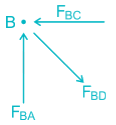At joint B, FBC → Compressive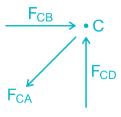At joitnt C, FCA → Tensile

Test: Structural Analysis- 3 - Question 2

### For a linear elastic frame, if the stiffness matrix is doubled with respect to the existing stiffness matrix, the deflection of the resulting frame will be

Detailed Solution for Test: Structural Analysis- 3 - Question 2

Stiffness (k) is defined as the force per unit deflection.

K = P/δ

⇒ δ = P/K

If stiffness matrix is doubled

i.e. K’ = 2K

then,

δ = P/K'

δ = P/2K

δ = (1/2)P/K

δ’ = δ/2.

Therefore, if the stiffness matrix is doubled with respect to the existing stiffness matrix, the deflection of the resulting frame will be half the existing value.

Test: Structural Analysis- 3 - Question 3

### The forces in members BE, CD, BC in the truss shown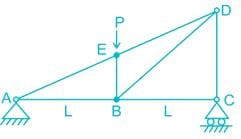Detailed Solution for Test: Structural Analysis- 3 - Question 3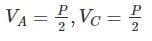At joint C, there is no horizontal reaction,

So, FBC=0,
Va = Vc = P
∴ Vc = P/2
∴ FCD = P/2
Now at joint E,

∑Falong the line BE = 0

FBE = P KN

Test: Structural Analysis- 3 - Question 4

Stiffness coefficient k23 for the beam shown below is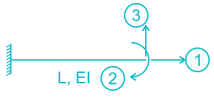Detailed Solution for Test: Structural Analysis- 3 - Question 4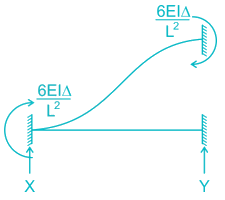For k33 Apply unit displacement along 3 and restraining the displacement in 2 and 1
Δ → unity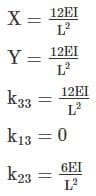Test: Structural Analysis- 3 - Question 5

Length of each member of the truss shown in the figure is 3m. Member AB and CD are subjected to a temperature rise of 40°C and coefficient of thermal expansion, α is 12 × 10-6/°C. The vertical deflection at joint E is________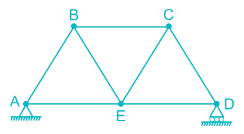Detailed Solution for Test: Structural Analysis- 3 - Question 5

To find the vertical deflection at joint E, a unit vertical load is applied at joint E and the forces in member AB and CD are to be found out.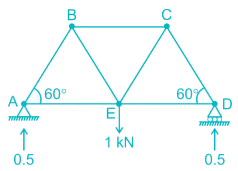Consider joint A,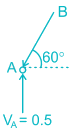FAB sin 60° = 0.5

FAB = 0.577 (compressive)

Similarly, FCD = 0.577 (compressive)

due to increase in temperature, the increase in length of AB & CD = L ∝ t

= 3 × 12 × 10-6 × 40

= 0.00144 m

So the deflection of joint E,

δ = Σ kδ’

where, k = Force produced in each member when a unit downward load is applied at joint E.

δ’ = Deflection produced in the concerned member (AB & CD)  due to temparature rise.

= (- 0.577) × (0.00144) + (- 0.577) × (0.00144)

= - 0.00166 m

= 1.66 mm (upward)

[‘–’ means our assumed downward deflection is wrong, it will deflect upward]

Test: Structural Analysis- 3 - Question 6

Uniformly distributed load w per unit length is suspended from a cable between point A and B. If the points A and B are at the same level at distance 'l' and central sage of the cable is h, the horizontal thrust developed of supports is -

Detailed Solution for Test: Structural Analysis- 3 - Question 6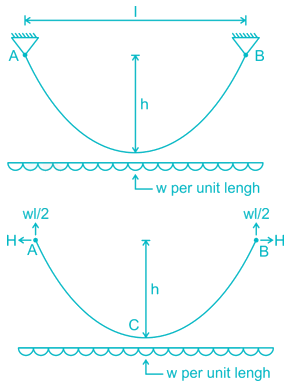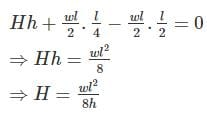Test: Structural Analysis- 3 - Question 7

Find the ration of the support moments, MA : MB (flexural rigidity is constant)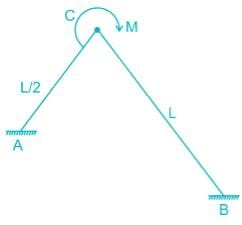Detailed Solution for Test: Structural Analysis- 3 - Question 7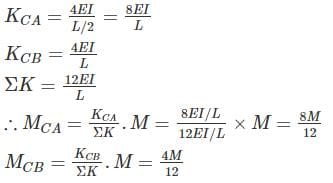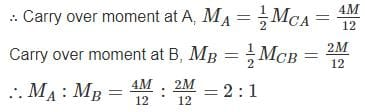Test: Structural Analysis- 3 - Question 8

A plane truss is shown below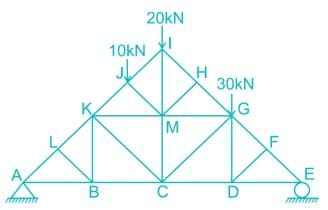The members who do not carry any force are:

Detailed Solution for Test: Structural Analysis- 3 - Question 8

I. Member LK, LA and LB are meeting at joint ‘L’ and AL and LK re co-linear so force in the member LB is zero.

FLB = 0

II. Member FG, FE, and FD are meeting at joint F, So force in the member FD = 0

FFD = 0

III. Removing Member LB, DF, thus member BK, BC and BA meets at point B out of which BA and BC are co-linear and vice versa for member DG, DC and DE.

So, FBK = 0

FDG = 0

IV. Similarly, Member HM, HI and HG are meeting at joint H

FHM = 0

Test: Structural Analysis- 3 - Question 9

The stiffness matrix of a beam element is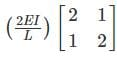Which one of the following is its flexibility matrix?

Detailed Solution for Test: Structural Analysis- 3 - Question 9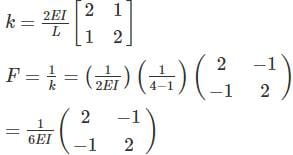Test: Structural Analysis- 3 - Question 10

A cable carrying a load of 20 kN/m run of the horizontal span, is structured at supports 100 m apart. The supports are at the same level and the central dip is 12.5 m. Find the least tension in the cable.

Detailed Solution for Test: Structural Analysis- 3 - Question 10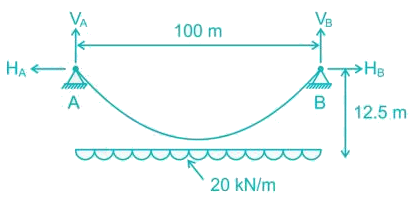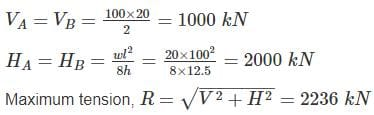Minimum tension occurs at deepest point and is equal to horizontal thrust.

Tmin = H = 2000 kN

## GATE Civil Engineering (CE) 2023 Mock Test Series

26 docs|292 tests
 Use Code STAYHOME200 and get INR 200 additional OFF Use Coupon Code
Information about Test: Structural Analysis- 3 Page
In this test you can find the Exam questions for Test: Structural Analysis- 3 solved & explained in the simplest way possible. Besides giving Questions and answers for Test: Structural Analysis- 3, EduRev gives you an ample number of Online tests for practice

## GATE Civil Engineering (CE) 2023 Mock Test Series

26 docs|292 tests